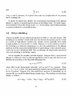# Plasma - Debye Shielding

• TheDestroyer
In summary, the conversation is about discrepancies between the results in a paper and the results obtained by the speaker in a PDF file. They discuss the possibility of using an infinite series to expand exponential terms and how it may affect the results. The speaker asks for clarification on the difference between their results and the results in the paper.

#### TheDestroyer

Hi Guyz,

Why the results in the paper I've attached aren't like the results I got in my pdf?

Please look at the paper in the last line, the second term doesn't correspond to what I calculated, What i calculated is in the pdf file, is it wrong?

Why in my work there is exponential terms while it's not in the paper?

#### Attachments

•p0009.jpg
39.1 KB · Views: 529
• ns.pdf
137.2 KB · Views: 224
Expand the exponential in terms of an infinite series, and ignore higher order terms, e.g. 1/T2 and higher, which one can do with reasonable accuracy for small arguments.

What you said might be correct if the terms are added, but they are subtracted, that means this will happen

e^x=1+x+0.5 x^2
e^-x=1-x+0.5 x^2

(e^x)-(e^-x)=(1+x+0.5 x^2)-(1-x+0.5 x^2)=((((2x))))

That means a linear term value will remain and must be multiplied in the term, Right? and this doesn't agree with the paper :(

Thank you anyway,

Any other ideas?

I believe if one uses the fact that ei = -ee = e, then the second term is multiplied by -1, and

e (e^x) - -e (e^-x) = e (1 + x + . . . ) + e (1 - x + . . .) = 2e (x), where

x = -e ($$\delta\phi$$) / T

and the expression becomes

-2 e2 ($$\delta\phi$$) / T

as in the paper or text.

Mr. Astronuc, Can you please check what I've written in the PDF?

if we did as you said then there will be more items multplied in the term, can you tell me what's the problem in the PDF? why it's not like the paper if we expanded the exponential terms in series?

Thank you